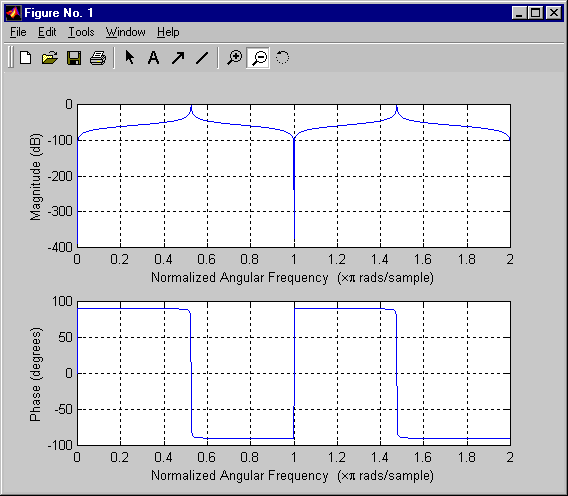EECS20N: Signals and Systems

# Evaluating Filter Quality

Consider the plot below comparing three filters:

If you had a java-enabled browser, you would see an applet here.

The differences between these filters are mainly in their stopband attenuation. Comparing filters in the stopband can be difficult because the gain in the stopband is typically very small compared to the gain in the passband. The comparison is easier to make if the frequency response is plotted using a logarithmic scale.

It is customary in electrical engineering to use decibels to get a logarithmic scale. Given a gain G, which is unitless (it is the ratio of two magnitudes with the same units), we can express it in decibels as

GdB = 20 log10(G).

This has units of decibels, written dB. The same frequency response as above is given below in decibels:

If you had a java-enabled browser, you would see an applet here.

Notice that the Matlab `freqz` function gives the frequency response in decibels: